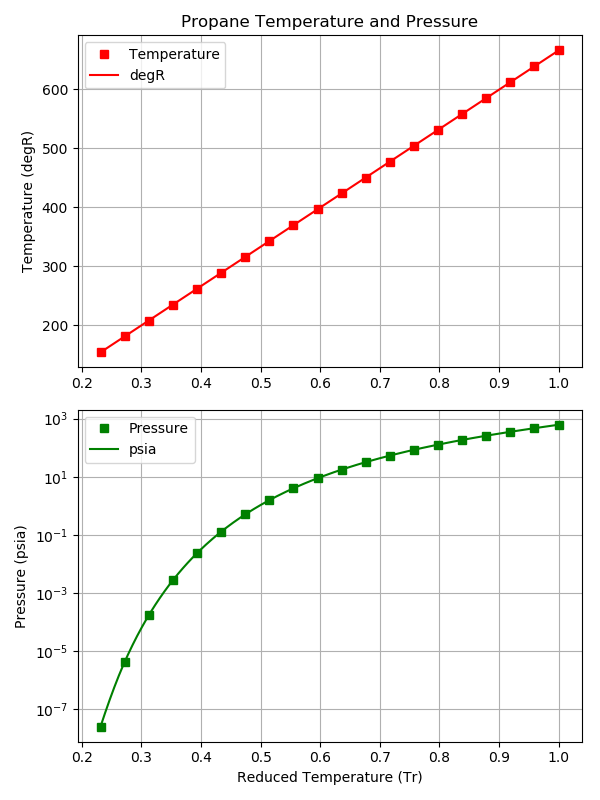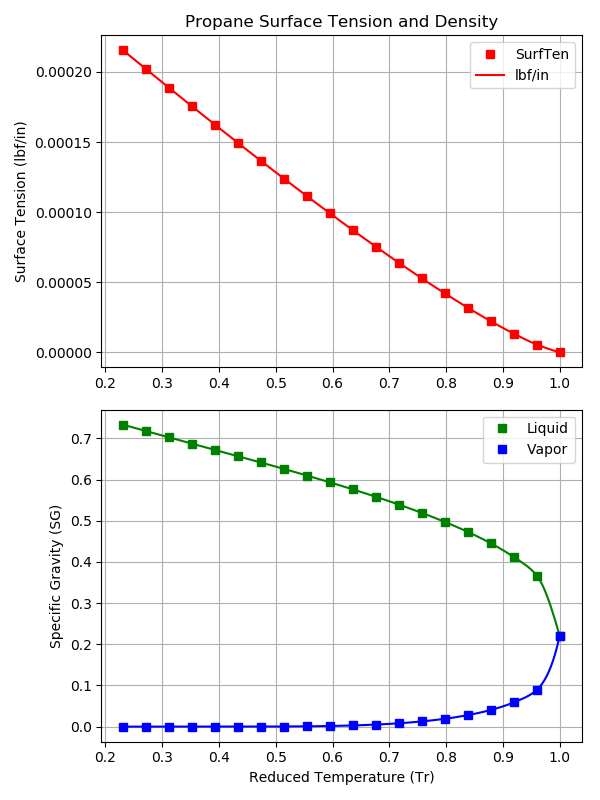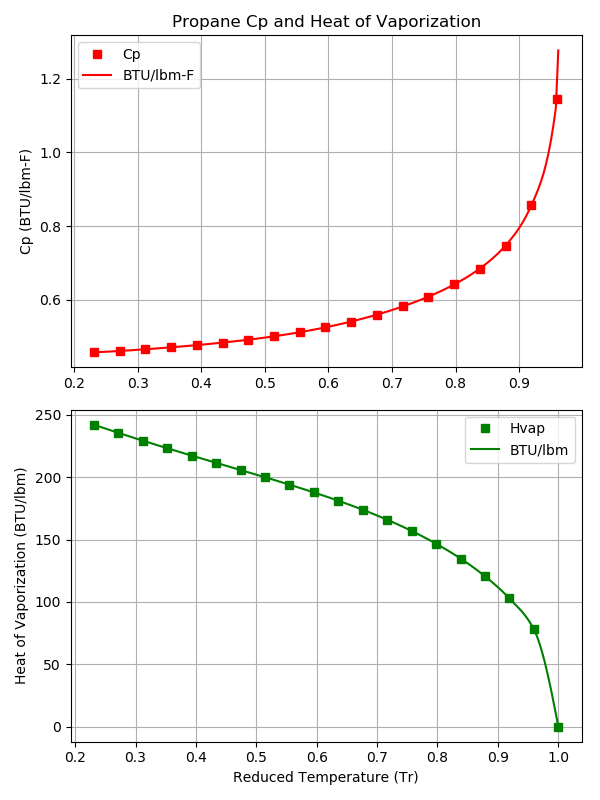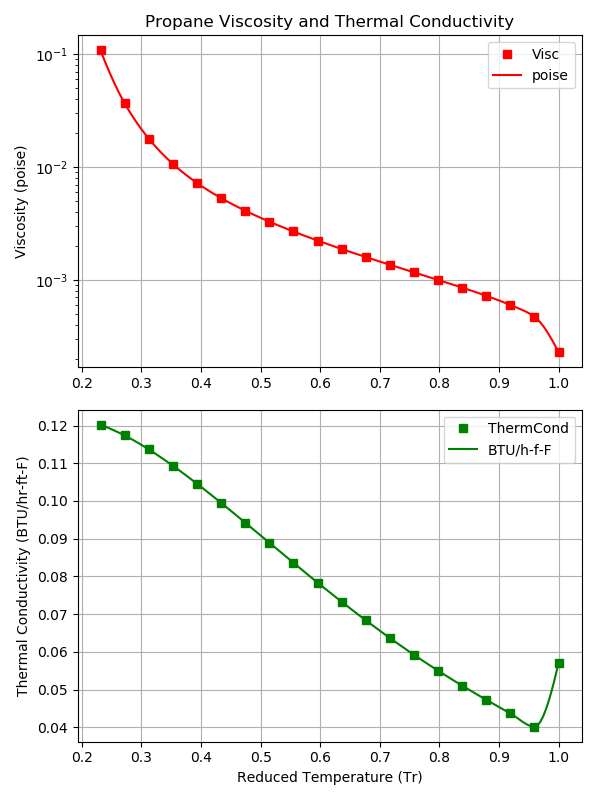# QuickStart¶

## Install RocketProps¶

The recommended way to install RocketProps is:

pip install rocketprops

OR on Linux
sudo pip install rocketprops
OR perhaps
pip install --user rocketprops


Note that RocketProps relies on scipy which, in turn, depends on numpy and matplotlib.

## Installation From Source¶

Much less common, but if installing from source, then pip is still a good option.

After navigating to the directory holding RocketProps source code, do the following:

cd full/path/to/rocketprops
pip install -e .

OR on Linux
sudo pip install -e .
OR perhaps
pip install --user -e .


This will execute the local setup.py file and install RocketProps.

## Running RocketProps¶

Test the installation by copying and pasting the following terminal command:

python -c "from rocketprops.rocket_prop import get_prop; p=get_prop('N2O4'); p.summ_print()"


The output should be a table of properties like the following:

====== RocketProps State Point of Liquid N2O4 =====
Name    =       N2O4  (MON-3, MON3)
T       =       527.67 degR
P       =      14.6959 psia
Pvap    =      13.7843 psia
Pc      =       1441.3 psia
Tc      =       776.47 degR
SGliq   =      1.44144 g/cc
SGvap   =   0.00367439 g/cc
visc    =   0.00420093 poise
cond    =    0.0766961 BTU/hr/ft/delF
Tnbp    =       530.07 degR
Tfreeze =       471.42 degR
Cp      =     0.374677 BTU/lbm/delF
MolWt   =       92.011 g/gmole
Hvap    =        178.2 BTU/lbm
surf    =  0.000149673 lbf/in


## Create Quick Plots¶

The following example will generate plots for Propane (C3H8) of its various liquid properties as a function of reduced temperature (Tr = T/Tc).

from rocketprops.rocket_prop import get_prop

p = get_prop('c3h8')
p.plot_sat_props(save_figures=True)